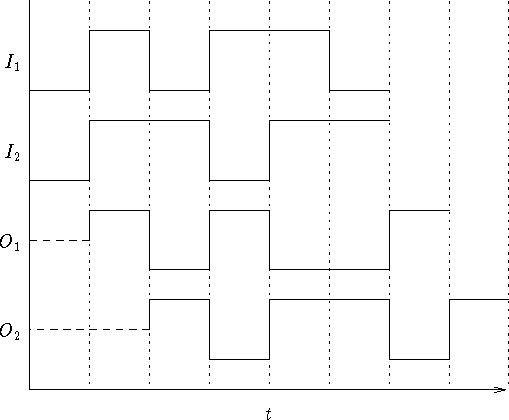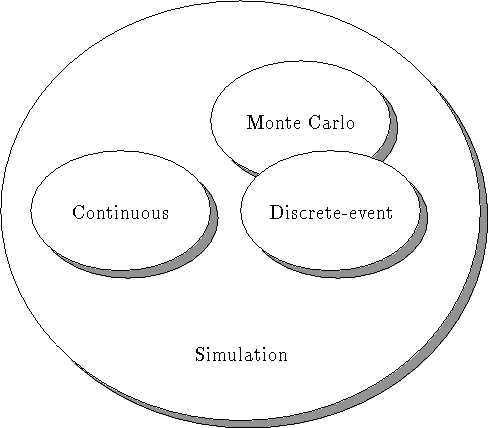Next: Abstraction Levels for Circuit Up: Simulation Models Previous: Continuous Simulation

## Discrete-Event Simulation

Discrete-event simulation  is used to simulate components which normally operate at a higher level of abstraction than components simulated by continuous simulators. Within the context of discrete-event simulation, an event is defined as an incident which causes the system to change its state in some way. For example, a new event is created whenever a simulation component generates output. A succession of these events provide an effective dynamic model of the system being simulated. What separates discrete-event simulation from continuous simulation is the fact that the events in a discrete-event simulator can occur only during a distinct unit of time during the simulation -- events are not permitted to occur in between time units. Discrete event simulation is generally more popular than continuous simulation because it is usually faster while also providing a reasonably accurate approximation of a system's behaviour.

As an example of discrete-event simulation, consider the logic circuit presented in Figure 1.3.Figure 1.3: Simple Digital Logic Circuit

Assuming that the NAND gate has a delay of two time units and the NOT gate has a delay of one time unit, the above logic circuit will produce the outputs given in Figure 1.4 when supplied with the specified inputs.Figure 1.4: Graph Representing Discrete Behaviour

Conventionally, a data structure known as a global event queue is used to process and manage the events and to activate components as required during the simulation. This report will demonstrate an improved technique for event management in which the global queue is eliminated in favour of distributed event queues. The design and implementation of such a queuing system is discussed in detail later in this report.

Monte Carlo simulation is related to discrete-event simulation. Monte Carlo simulators usually make extensive use of random number generators in order to simulate the desired system. Unlike discrete-event simulators, which are often used to model deterministic systems, Monte Carlo simulators can be used to effectively model systems in which probability and nondeterminism plays a major role. As such, Monte Carlo simulators are commonly used to model stochastic systems. The relationship between the three types of simulations is displayed in Figure 1.5.Figure 1.5: Simulation Models

Hierarchical simulation, although not a simulation type by itself, may be used in conjunction with continuous or discrete even simulators to simplify the simulation process. Hierarchical simulation is a process whereby higher order components delegate behavioural responsibility to its composite subcomponents. The higher level components are responsible for activating their respective child components in a meaningful sequence so as to model the correct behaviour of the system. As mentioned earlier, hierarchical design and simulation is one of the techniques used to cope with the complexity associated with a given system. Later chapters will demonstrate how the object-oriented paradigm lends itself very well to the description and simulation of hierarchical systems.Next: Abstraction Levels for Circuit Up: Simulation Models Previous: Continuous Simulation

Donald Craig
Mon Jul 8 12:05:35 NDT 1996